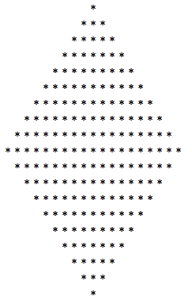# How to make a Star Polygon in PHP ?

We create a star polygon. Here we use eight ‘for’ loops. First and fifth are main and other are nested in these. First for loop is used for number of lines in upper triangle and fifth loop is used for number of lines in lower triangle.
Second for loop is used to print spaces, third loop is used for left side right angle triangle that will show stars according line number and fourth loop is used for right side right angle triangle that will show stars according to last line number.
Sixth loop is used to print spaces and seventh is used to show stars in reverse order in lower left triangle(right angle triangle).
Eighth is used to show stars in reverse order in right side triangle(right angle triangle).
Here \$n is used for number of lines in first loop and number of spaces in second loop.
syntax with output as below.

 ```1; \$c--) { echo "*"; } echo "
"; \$m++; } for(\$i=1; \$i<=5; \$i++) { for(\$j=\$i; \$j>=1; \$j--) { echo "  "; } for(\$k=\$n; \$k>1; \$k--) { echo "*"; } for(\$c=\$n-1; \$c>1; \$c--) { echo "*"; } echo "
"; \$n--; } ?>```